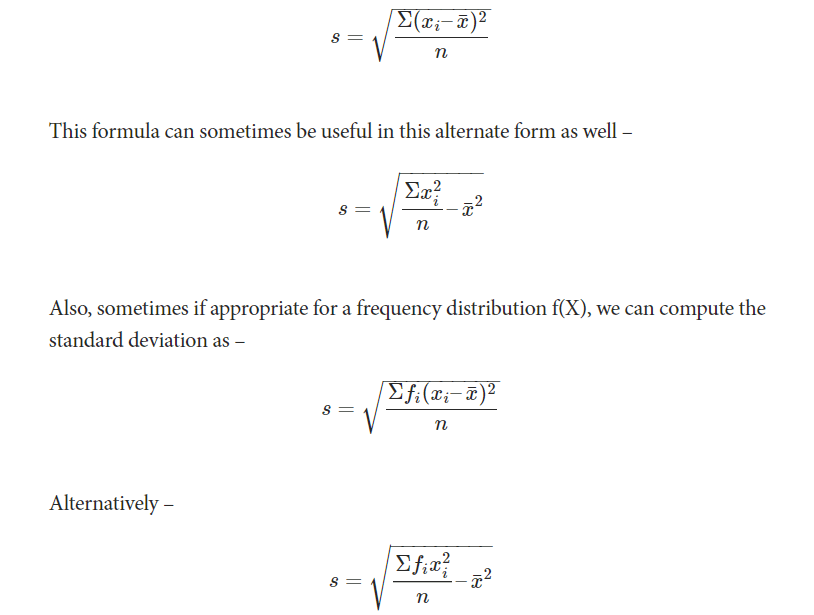Skip to content

# Mean Deviation and Standard Deviation

### Mean Deviation

To understand the dispersion of data from a measure of central tendency, we can use mean deviation. It comes as an improvement over the range. It basically measures the deviations from a value. This value is generally mean or median. Hence although mean deviation about mode can be calculated, mean deviation about mean and median are frequently used.

Note that the deviation of an observation from a value a is d= x-a. To find out mean deviation we need to take the mean of these deviations. However, when this value of a is taken as mean, the deviations are both negative and positive since it is the central value.

This further means that when we sum up these deviations to find out their average, the sum essentially vanishes. Thus to resolve this problem we use absolute values or the magnitude of deviation. The basic formula for finding out mean deviation is :

Mean deviation= Sum of absolute values of deviations from ‘a’ ÷ The number of observations

### Standard Deviation

As the name suggests, this quantity is a standard measure of the deviation of the entire data in any distribution. Usually represented by or σ. It uses the arithmetic mean of the distribution as the reference point and normalizes the deviation of all the data values from this mean.

Therefore, we define the formula for the standard deviation of the distribution of a variable X with n data points as:### 4 Comments »

error: Content is protected !!